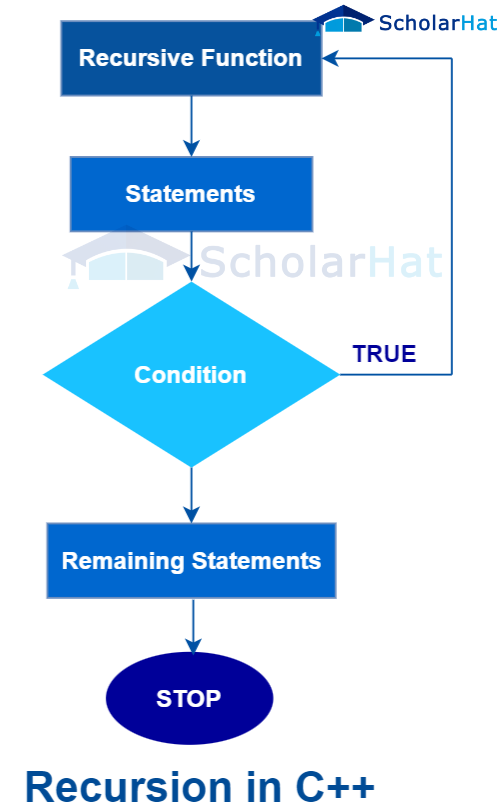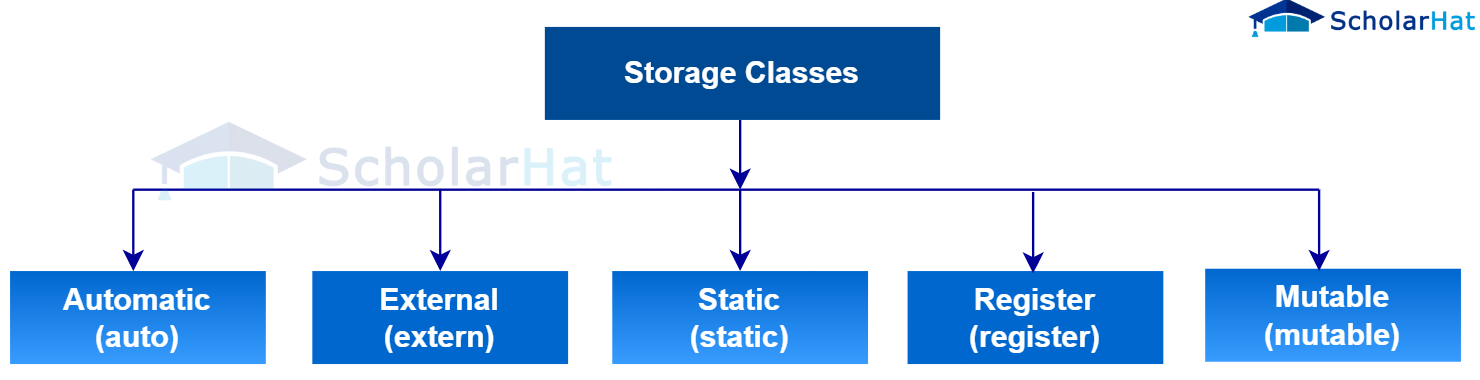# Recursion and Storage class in C++

16 Feb 2023
Intermediate
137 Views

## Introduction

Are you curious about the world of C++ and all it has to offer? Have you ever wondered how recursion and storage classes in C++ work together? In this blog post, we'll explore the ins and outs of both concepts so that you can learn more about the powerful programming language. You'll come away with an understanding of how code structures work, what recursion is all about, and a look into each of these storage classes along with their characteristics. Whether you're a beginner or an advanced user, by reading through this post, your understanding of C++ will be greatly increased!

## What is Recursion in C++

Recursion in C++ is a programming technique in which a function calls itself from within its own code. Recursive functions can allow for better scalability and organization of code, enabling greater readability. Recursion offers an advantage over iterative equivalents that also accomplish the same task, being easier to understand at first glance. It can be used for many applications including mathematical solutions and tree searches. Recursive programs should be structured well with ample tracking of progressions and provide clear stopping criteria, as errors can occur quickly when creating recursive functions. Implementing recursion in C++ can lead to sophisticated yet organized solutions with much less code than other methods while maintaining readability.Syntax

``````recursionfunction()
{
recursionfunction(); //calling self function
}
``````

Example

``````#include<iostream>
using class std;
int main()
{
int factorial(int);
int fact,value;
cout<<"Enter any number: ";
cin>>value;
fact=factorial(value);
cout<<"The factorial of a number is: "<<fact<<endl;
return 0;
}
int factorial(int n)
{
if(n<0)
return(-1); /*Wrong value*/
if(n==0)
return(1); /*Terminating condition*/
else
{
return(n*factorial(n-1));
}
}
``````

Output

``````Enter any number: 10
The factorial of a number is: 3628800
``````

## What is Storage class in C++

Storage class in c++ is generally used to define the visibility and lifetime of any variable or function in c++ programming including the scope of the program which will further help the programmer to understand the existence of any variable during the run time of the program. Storage class in C++ is a type of keyword that allows developers to manipulate the visibility and life cycle of program objects. Storage classes tell the compiler where to store variables, whether they will live on the stack or in the heap area, and what scope they have. Storage classes can also control which parts of an application have access to certain objects.

## Types of Storage Class in C++

There are 5 types of storage classes in c++:

1. Automatic Storage Class in c++
2. Register Storage Class in c++
3. Static Storage Class in c++
4. Extern Storage Class in c++
5. Mutable Storage Class in c++## Automatic Storage Class in c++

The automatic Storage Class in c++ programming provides the capabilities of type interface by using automatic deduction of the data type of aj expression in any programming language. This method takes less time to write the code, which is known by the compiler. The deductions of the types generally are done in the phase of compilation but it does not affect the run time of the program.

Example

``````#include <iostream>
using class std;
void autoStorageClass()
{
cout << "Demonstrating auto class\n";
// Declaring an auto variable
// No data-type declaration needed
auto A = 45;
auto B = 4.5;
auto C = "Scholarhat";
auto D = 'S';
// printing the auto variables
cout << A << " \n";
cout << B << " \n";
cout << C << " \n";
cout << D << " \n";
}
int main()
{
// To demonstrate auto Storage Class
autoStorageClass();
return 0;
}
``````

Output

``````Demonstrating auto class
45
4.5
Scholarhat
S
``````

## Static Storage Class in c++

This particular static storage class in c++ programming is used for the declaration of the static variables that are generally used to write programs in c++ language. Static variables have a property that helps to preserve the value even after they are out of their scope. So the program is initialized once and exists until the termination. So no new memory allocation is not needed because the program is not redeclared. The program is denied if its scope varies from local to function. The global static variables can be accessed from anywhere in the program.

Example

``````#include <iostream>
using class std;
// Function containing static variables
// memory is retained during execution
int staticFun()
{
cout << "For static variables: ";
static int count = 0;
count++;
return count;
}

// Function containing non-static variables
// memory is destroyed
int nonStaticFun()
{
cout << "For Non-Static variables: ";
int count = 0;
count++;
return count;
}
int main()
{
// Calling the static parts
cout << staticFun() << "\n";
cout << staticFun() << "\n";

// Calling the non-static parts
cout << nonStaticFun() << "\n";
cout << nonStaticFun() << "\n";
return 0;
}
``````

Output

``````For static variables: 1
For static variables: 2
For Non-Static variables: 1
For Non-Static variables: 1
``````

## Register Storage Class in c++

The register storage class in c++ programming generally declares the register variables with the same functionality as the auto variables. The only difference they have is that the compiler tries to store the variables in the register of the designated microprocessor if the register is available. It makes the register variable work faster to store in memory during the run time of the c++ program.

Example

``````#include <iostream>
using class std;
void registerStorageClass()
{
cout << "Demonstrating register class\n";
// declaring a register variable
register char a = 'S';
// printing the register variable 'a'
cout << "Value of the variable 'a'"
<< " declared as register: " << a;
}
int main()
{
// To demonstrate register Storage Class
registerStorageClass();
return 0;
}
``````

Output

``````Demonstrating register class
Value of the variable 'a' declared as register: S
``````

## External Storage Class in c++

External storage class in c++ programming identifies the variables as defined elsewhere and not saved within the block. The value that is assigned to it is in a different block and can be changed into a different block.

Example

``````#include <iostream>
using class std;

// declaring the variable which is to
// be made extern an initial value can
// also be initialized to y
int y;
void externStorageClass()
{
cout << "Demonstrating extern class\n";
// telling the compiler that the variable
// y is an extern variable and has been
// defined elsewhere (above the main
// function)
extern int y;
// printing the extern variables 'y'
cout << "Value of the variable 'y'"
<< "declared, as extern: " << y << "\n";
// value of extern variable y modified
y = 3;
// printing the modified values of
// extern variables 'y'
cout
<< "Modified value of the variable 'y'"
<< " declared as extern: \n"
<< y;
}
int main()
{
// To demonstrate extern Storage Class
externStorageClass();
return 0;
}
``````

Output

``````Demonstrating extern class
Value of the variable 'y' declared, as extern: 0
Modified value of the variable 'y' declared as extern:
3
``````

## Mutable Storage Class in c++

A mutable storage Class in c++ programming is used for modifying one or more data members of a structure through a constant function. This task can easily be promoted by Mutable keywords which are particularly used to allow a particular data member of an object to be a Modifier.

Example

``````#include <iostream>
using std::cout;
class Test
{
public:
int a;
// defining mutable variable b
// now this can be modified
mutable int b;
Test()
{
a = 4;
b = 10;
}
};
int main()
{
// t1 is set to constant
const Test t1;
// trying to change the value
t1.b = 20;
cout << t1.b;
// Uncommenting below lines
// will throw error
// t1.a = 8;
// cout << t1.a;
return 0;
}
``````

Output

``````20
``````

## Summary

Recursion is a process of repeating items in a self-similar way. Storage class defines the scope and lifetime of variables and/or functions within a C++ program. In this article, we looked at how recursion works in C++ and how storage classes help to define the scope and lifetime of variables. We also saw how automatic, register, static, mutable, and extern storage classes work with examples. They allow you to control the visibility and lifetime of variables, which can be very useful in larger programs. Thanks for reading! I hope this article was helpful in understanding these two concepts.